Science Journal of Applied Mathematics and Statistics
Volume 3, Issue 3, June 2015, Pages: 136-143

Empirical Research on VAR Model Based on GJR-GARCH, EVT and Copula

Hong Zhang1, Li Zhou1,Shucong Ming2, Yanming Yang3, Mengdan Zhou4

1School of Information, Beijing Wuzi University, Beijing, China

2Chinese Academy of Finance and Development, Central University of Finance and Economics, Beijing, China

3Information Technology Department, East China Institute of Technology, Nanchang, China

4School of Media Studies and Humanities, Zhejiang University City College, Hangzhou, China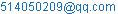(Hong Zhang)

Hong Zhang, Li Zhou, Shucong Ming, Yanming Yang, Mengdan Zhou. Empirical Research on VAR Model Based on GJR-GARCH, EVT and Copula. Science Journal of Applied Mathematics and Statistics. Vol. 3, No. 3, 2015, pp. 136-143. doi: 10.11648/j.sjams.20150303.16

Abstract: In this paper, we establish GJR-GARCH models to extract the residuals of logarithmic returns of two index--- New York stock exchange composite index (NYA) and NASDAQ. and estimate the distribution function of the residuals utilizing Gaussian kernel method and Extreme Value Theory. The kernel cumulative distribution function estimates are well suited for the interior of the distribution where most of the residuals are found and the POT method of Extreme Value Theory fits the extreme residuals in upper and lower tails well. The monte carlo technique is used to simulate the income of securities index 20000 times after we get the marginal distribution of the residual income of securities index. Secondly, By using the copula function to get the joint distribution of mthe two stock index. Lastly, According to the theory of VAR calculate VAR value of the portfolio consisting of two equal weight comprehensive index in different confidence levels.

Keyword: Extreme Value Theory, VAR Model, GJR-GARCH

1. Introduction

In this paper, we study VaR model and its over-arching theories including FHS technology, GARCH model, Copula theory, Extreme Value Theory, etc, which are widely applied in describing, fitting and forecasting the financial time series as an effective and efficient approach to the evaluation and measure of the risk pertaining to the financial assets. This paper approximately consists of three empirical researches and simulations as follows:

In the third place, we construct GJR-GARCH models to extract the residuals of logarithmic returns of stock indices, and estimate the distribution function of the residuals utilizing Gaussian kernel method and Extreme Value Theory. The kernel cumulative distribution function estimates are well suited for the interior of the distribution where most of the residuals are found and the POT method of Extreme Value Theory fits the extreme residuals in upper and lower tails well. Results show that during the holding period (one day) the greatest loss of this portfolio is 12.6225% and the greatest return rate is 8.9172%. The VaR values are -1.6377%,-2.2782%and-4.2981% under the confidence level of 90%, 95% and 99% respectively, namely we have 90% possibility that the loss is less than 1.6377%, have 95% possibility that the loss is less than 2.2782% and have 99% possibility that the loss is less than 4.2981%1-5.

2. The Empirical Analysis

2.1. The Selection of Data and Its Characteristics

Selecting New York stock exchange composite index(NYA) and NASDAQ as research object. Sample interval is from 2003.1.4 to 2014.12.30. Using Logarithm yields,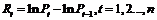.

The P is the closing price of stock index at time t. The essential information is below.

Known from figuer 3, Distritution of daily logarithmic return of NYA and NASDAQ are fat-tail on both ends of gains and losses. and JB- statistic is significantly. So the results refuse the hypothesis of normal distribution.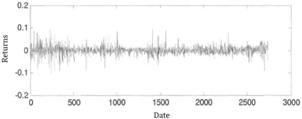Figure 1. Daily logarithmic return of NYA.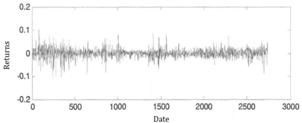Figure 2. Daily logarithmic return of NASDAQ.

Table 1. Statistical indicators of NYA and NASDAQ.

 Name Mean STD Slewness Kurtosis JB- statistic NYA 0.00068 0.0167 -0.0701 9.2469 4052.248 NASDAQ 0.00081 0.01814 -0.3541 8.0148 3978.415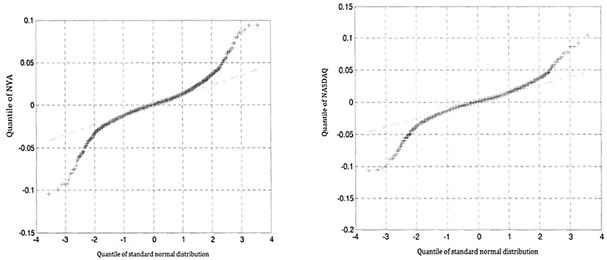Figure 3. Normal Q-Q Plot of daily logarithmic return of NYA and NASDAQ.

2.2. The Establishment of the Model and the Analysis of the Results

Autocorrelogram and partial- autocorrelogram of the logarithmic return suggest that yield sequence presents a certain degree of serial correlation, Further, autocorrelogram of the square of the logarithmic return suggest that yield sequence square presents serial correlation significantly6-9.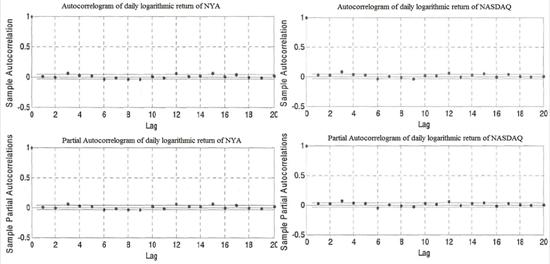Figure 4. Autocorrelogram and partial- autocorrelogram of daily logarithmic return of NYA and NASDAQ.

The paper adopt Student-t distribution to estimate residuals instead of normal distribution. Next we estimate six ARMA-GJR-GARCH models. (table 2)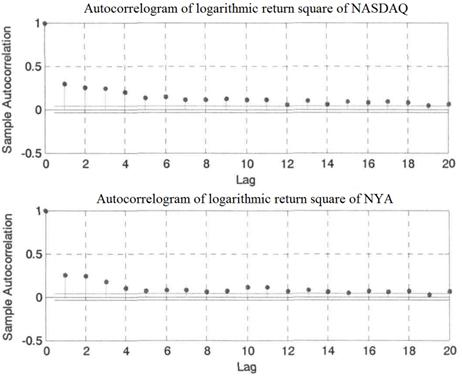Figure 5. Autocorrelogram of logarithmic return square of NYA and NASDAQ.

Table 2. Parameters estimation & Significant level& Evaluation index of the models.

 Parameters The models of NYA Model 1 Model 2 Model 3 Model 4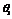-0.7677 ( 0.0000 ) 0.1475( 0.0000 )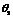0.8571( 0.0000 )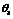0.07763 ( 0.0000 ) 0.0761 ( 0.0000 )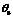-0.0470 ( 0.0106 ) -0.0474 ( 0.0079 )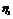0.7988 ( 0.0000 ) -0.1148( 0.0000 )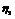-0.8772( 0.0000 )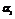0.0894 ( 0.0065 ) 0.0825 ( 0.0019 ) 0.1105 ( 0.0000 ) 0.0724( 0.0007 )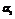0.0217 ( 0.005257 ) -0.0599 ( 0.0340 ) 0.0621( 0.0101)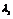0.0750 ( 0.0120) 0.1985 (0.0010) 0.0734 ( 0.0114 ) 0.1240( 0.0018 )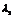-0.1946 ( 0.0007 ) -0.0830( 0.0275 )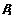0.8265 ( 0.0000 ) 1.5581 ( 0.0000 ) 0.8291 ( 0.0000 ) 0.7805( 0.0000 )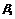-0.5864 (0.0000) SSR 0.7682 0.7682 0.7723 0,7733 AIC -5.6985 -5.6929 -5.6822 -5.6454 SBC -5.6712 -5.671 -5.6671 -5.6216 Q(4) 6.5877(0.0371) 8.4594 (0.0146) 11.0605 ( 0.0040 ) 6.0072 Q(8) 8.8214(0.1839) 10.3973 (0.1089) 15.4999 (0.0167) 11.7042 (0.0197) Q(12) 15.4322(0.1171) 16.6584(0.0823) 24.6131 (0.0061 21.1750 (0.0067) Q(4) 1.9467(0.3778) 0.9806(0.6125) 1.3057 ( 0.5206 ) 0.9659 Q(8) 5.2070(0.5175) 2.3949(0.8800) 4.7139 (0.5810) 5.2802 (0.2597) Q(12) 6.3938 (0.7812) 4.1401(0.9408) 6.0026(0.8150) 6.0731 ( 0.639 )

According to SSR Parameters significantly AIC and SBC determine the final model . The SSR of model 1 and model 3 are smaller than the SSR of model 2; but Q-statistic of model 3 and model 2 both significant suggest that there are still some residual serial correlation. while Q-statistic of model 1 is no significant, suggesting that the mean model and variance model are both great. And according to the principle of AIC and SBC, we chose the model whose AIC and SBC are minimum, that is model 1. Accordingly, Considering the NASDAQ, The SSR of model 4 and model 6 are smaller than the SSR of model 5; but Q-statistic of model 4 significant suggest that there is still some residual serial correlation. while Q-statistic of model 4 and model 6 are no significant, suggesting that the mean model and variance model are both great. And according to the principle of AIC and SBC, we chose the model whose AIC and SBC are minimum, that is model 410-12.

Two models ‘concrete form established in view of the two securities index as below

The conditions average model of the model 1 is seasonal ARMA model: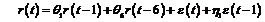(1)

Asymmetric conditional heteroscedastic model for model 1 is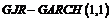: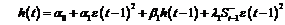(2)

where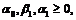(3)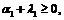(4)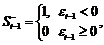(5)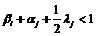(6)

The conditions average model of the model 4 is seasonal ARMA model:(7)

Asymmetric conditional heteroscedastic model for model 4 is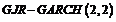:(8)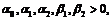(9)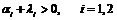(10)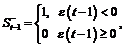(11)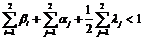(12)

The Seasonal ARMA model is adopted in the paper to get residual series of NYA and NASDAQ; and conditional standard deviation filtering of NYA and NASDAQ are obtained with GJR-GARCH. (Figure 6)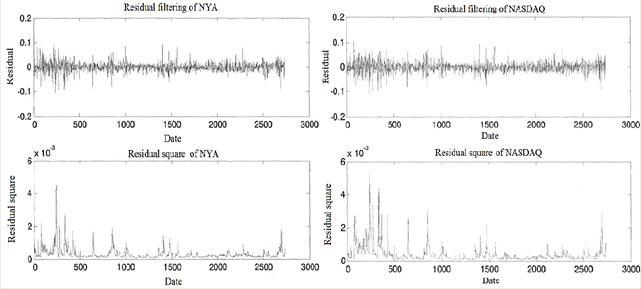Figure 6. Residual filtering and Residual square of NYA and NASDAQ.Figure 7. Autocorrelogram of standard error and its square of NYA and NASDAQ.

We obtained the standardization, and satisfies the independence with the distributed residual, then using the Gaussian kernel function estimated that two kinds refer to the number the experience cumulative distribution function, this method causes to present the stepped the cumulative distribution function becomes smooth. Fits function the thought are as follows using the Gaussian kernel function13-14: using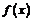to fit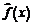.

Where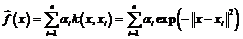(13)

The method of Gauss nucleus estimate may very good estimate internal distribution function, but regarding two estimate effects is not very ideal. For better estimate two distributions, this article to falling into two standardized residuals applies POT that in EVT developed in recent years (Peaks Over Threshold) model.

The POT model to observing in the value all surpasses some big threshold value (Threshold) data modeling. Because the POT model has used the limited violent observation value effectively, therefore is generally considered that in reality is most useful. Establishes the tail and under the threshold value of tail, enables two to contain 10% residuals respectively, then with the method that the maximum likelihood estimated that the generalized Pareto distribution fits exceeds the threshold value the data. We got on the NYA and NASDAQ and lower thresholds for threshold as shown in table 3. Table 4 estimated values for generalized Pareto distribution parameters. Figure 8 shows the experience in NYA and NASDAQ Exchange composite distribution, of which two are made to fit the generalized Pareto distribution, Interior is fitted with Gaussian kernel density estimation method. We test whether the generalized Pareto distribution of simulated NYA and NASDAQ and composite two-tailed, and by Figure 9 generalized Pareto distribution can be a good simulation of log earnings residual two-tailed Distributions.

Table 3. Pareto distribution parameters.

 NYA NASDAQ The threshold value 78.1784 79.1672 The lower value -80.2183 -84.3438

Table 4. Pareto distribution parameters.

 Pareto distribution parameters NYA NASDAQ Top end Bottom end Top end Bottom end Shape parameter 0.04971 0.16341 0.10944 0.09537 Scale parameter 47.97077 45.24693 37.90079 48.63171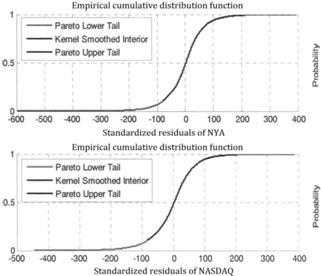Figure 8. Empirical cumulative distribution function of NYA and NASDAQ.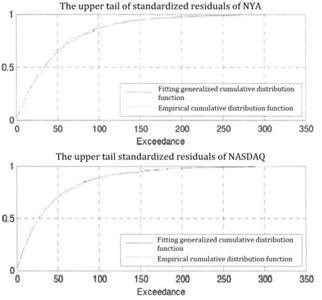Figure 9. Pareto distribution function and empirical distribution fitting text.

Application of Monte Carlo simulation based on Student-t Copula have to identify two indexcorrelation coefficient matrix of the residuals and the degrees of freedom.

Nystrom and Skoglund put forward the degrees of freedom provided as researchers custom analog input, to allow researchers to subjective pushed to the tail dependence of the related assets. In particular, they set up a relatively small degree of freedom, for example, 1,2, in order to be able to have a detailed inspection of possibility of occurrence of the associated extreme. This approach is different from normal distribution assumptions usually.

It is extremely important for traditional VAR analysis and stress testing of extremism of dependency measure.

The relationship of Spearman rank correlation coefficient and the linear correlation coefficient in the Student function is as follows: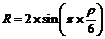(14)

To compare residual error of the two index and Q-Q map of Student distribution, found that NYA and NASDAQ gains residuals and student distribution whose df is 3 are most closely. Therefore, this paper will set degrees of freedom at three. In order to apply Student-t Copula method, we should get each of the required parameters.

First calculating the Spearman rank correlation coefficient; We can obtain the linear correlation coefficient by using the standard sin function transformation of the Spearman rank correlation coefficient. Marginal distributions of two random variables are subject to the freedom of Student-t distribution and the correlation coefficient is r. Therefore we can take advantages of Student-t Copula Methods to produce 4000 random numbers obeying that the freedom is 3 and the linear correlation coefficient is r. Following, this 40,000-random numbers will be converted into interrelated uniformly distributed random variables by cumulative distribution function of t distribution.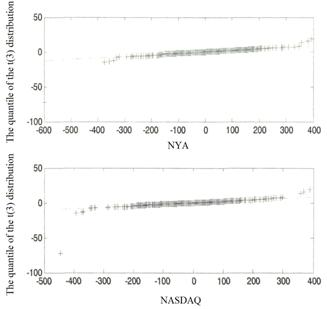Figure 10. The quantile of the t(3) distribution of NYA and NASDAQ.Figure 11. Associated residuals simulation by t(3) copulas.

We've got the residuals which are associated with t (3) Copula simulation, and through the ARMA - GJR - GARCH model, model 1 and model 4 predict the yield of NYA and NASDAQ, getting 20000 combinations of the yield of NYA and NASDAQ. In this paper, we establish an equal weighted portfolio which is composed of NYA and NASDAQ, and the weight of two assets both are 0.5.We assume that the weights of the portfolio in the holding period (24 hours) are fixed, and it means that it is a process of self financing, and we do not consider the transaction cost in this paper. The simulation results are shown in table 5: the portfolio in the holding period (24 hours) has the maximum loss of 12.6225%, and its maximum possible gain is 8.9172% ,and while the confidence level is 90%, 95% and 99%, the VaR is -1.6377%, -2.2782% and -4.2981%,respectively, in the other words, there is a 90% possibility of the loss not more than 1.6377% , and a 95% possibility of the loss not more than 2.2782%, and a 99% possibility of the loss not more than 4.2981%. Figure 11 is a Mont Cartlo simulation of investment combination’s logarithm cumulative distribution of benefits when the holding period is one day.

Table 5. The simulation results.

 confidence level 90% 95% 99% VaR 1.6377% 2.2782% 4.2981% Simulation of the maximum loss 12.6225% Simulation of the maximum return 8.9174%Figure 12. simulation of the holding period is 1 day portfolio cumulative distribution of the logarithmic benefit.

3. Summary

This paper is to extract the residual of two stock index logarithm return by establishing ARMA-GJR-GARCH model with seasonal adjustment. According to the model test, the estimated ARMA-GJR-GARCH model can not only eliminate the ARCHIGARCH effect of logarithm return sequence and fit sample data better, but also can show the entire dynamic behaviors of the mean model and variance model. The estimation of the distribution of residuals using Gaussian kernel estimation and extreme value theory (EVT) is ideal. The former can estimate the internal distribution function commendably and the latter can have a good two tails estimation of the distribution function. We have simulated the stock index return for 20,000 times wielding Monte Carlo techniques, thus Copulas function are used to get the joint distribution of two stock indexes. Accurate estimating the marginal distribution of the two securities index return guarantees to get reliable joint distribution of securities index function by Copulas function. According to the VaR theory, we calculated the portfolio VaRs under different confidence levels that the portfolio is composed of two equal weighted comprehensive indexes. During the holding period (24 hours),the maximum loss of this portfolio is12.6225%, the maximum possible gains is 8.9172%.The VaR value are 1.6377%, 2.2782% and 4.2981% when the confidence levels are 90%, 95% and 99% respectively. GJR-GARCH, EVT and Copulas combination model can well capture the complex correlation structure among the financial market events which will be widely used in the field of financial risk management, early warning and control.

Acknowledgements

This project (Empirical research on Stock index investment risk model, No.68) is funded by the "2014-2015 school year, Beijing Wuzi University, College students' scientific research and entrepreneurial action plan project". And by Beijing Wuzi University, Yunhe scholars program(00610303/007).And by Beijing Wuzi University, Management science and engineering Professional group of construction projects. (No.PXM2015_014214_000039)

References

1. Andrey I. Kibzun, Evgeniy A. Kuznetsov.Analysis of criteria VaR and CVaR,.Nournal of Banking&Finance,2006(30):779-796.
2. Le Lei, Sulin Pang, An Empirical Research on the Chinese stock market based on 'VaR, 2007 IEEE International Conference on Control and Automation, 2007:2729-2734.
3. Yau Man Zeto SamueLValue at risk and conditional extreme value theory via mark:ov regime switching models, The Journal of Futures Markets, 2008(28):155-181.
4. Alexandra Costello, Ebenezer Asem, Eldon Gardner. Comparison of Historically Simulated VaR: Evidence from Oil Prices, Energy Economics, 2008(10):1600-1623.
5. Allan Gregory, Jonathan Reeves. Interpreting value at risk (VaR) forecasts, 2007(3):1-20.
6. Malay Bhattacharyya, Gopal Ritolia. Conditional VaR using EVT Towards a planned margin scheme, International Review of Financial Analysis, 2008(17):382-395.
7. Michael Mcaleer, Bernardo Do Veiga. Forecasting Value-at-Risk with a Parsimonious Portfolio Spillover GARCH(PS-GARCH) Model, Journal of Forecasting, 2008(27):1-19.
8. Jenkinson A F. The frequency distribution of the annual maximum(or mimimum) values of meteorological elements, Quarterly Journal of the Royal meteorological society,1955(81):145-158.
9. Christian Genest,Jock MacKay. The Joy of Copulas: Bivariate Distributions with Uniform Marginals, The American Statistician, 1986(40):280-283.
10. Joe H, Multivariate Models and Dependence Concepts. 2004: Chapaman&Hall.
11. Umberto Cherubim, Elisa Luciano, Walter Vecchiato, Copula Methods in Finance;. 2004: John Wiley&Sons.
12. MingHeng Zhang,QianSheng Cheng, An approach to VaR for capital markets with Gaussian mixture, Applied Mathematics and Computation, 2005(168):1079-1085.
13. Dennis Bams, Thorsten Ixhnert, Christian C.P. Wolff. An evaluation framework for alternative VaR-models, Journal of International Money and Finance, 2005(24):944-958.
14. Matthew Pritsker, The Hidden Dangers of Historical Simulation, Journal of Banking&Finance, 2006(30):561-582.

 Contents 1. 2. 2.1. 2.2. 3.
Article ToolsAbstractPDF(737K)